# 吉布斯Gibbs 采样器

Gibbs 采样器是 Metropolis-Hastings 采样器的一个特例，通常在目标是多元分布时使用。

Gibbs 采样器生成马尔可夫链如下：

• 让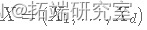是 Rd 中的随机向量，在时间 t=0 初始化 X(0)。
• 对于每次迭代 t=1,2,3,…重复：

• 设置 x1=X1(t-1)。
• 对于每个 j=1,…,d：
• 生成 X∗j(t) 从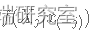， 其中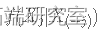是给定 X(-j) 的 Xj的单变量条件密度。
• 更新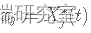.
• 当每个候选点都被接受时，设置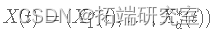.
• 增加 t。

λ 的完整条件分布由下式给出：

α 的完整条件分布由下式给出：

k 的完整条件分布由下式给出：

# 计算方法

## 数据

set.seed(98712)
y <- c(rexp(25, rate = 2), rexp(35, rate = 10))

## Gibbs采样器的实现

n <- length(y) # 样本的观察值的数量
lci <- 10000 # 链的大小
aba <- alpha <- k <- numeric(lcan)
k$1$ <- sample(1:n,

# 现在，对于算法的每次迭代，我们需要生成 λ(t)、α(t) 和 k(t)，如下所示（记住如果 k+1>n 没有变化点）：

for (i in 2:lcan){
kt <- k$i-1$
# 生成lambda
lambda$i$ <- rgamma
# 生成α
# 产生k
for (j in 1:n) {
resfil

# 结论

Kaizong Ye拓端研究室（TRL）的研究员。

​非常感谢您阅读本文，如需帮助请联系我们！QQ在线咨询

15121130882

0571-63341498

## 关注有关新文章的微信公众号

This will close in 0 seconds